Have you ever wanted to try to swap values from one cell to another? It is possible using Macros in Excel!

Plus you also get to learn some programming along the way, how cool is that?

Make sure your Excel has the Developer Tab enabled following this tutorial.

Here are the 2 values that we want to swap: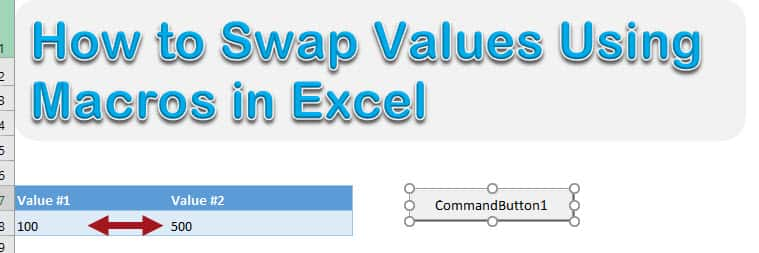I will show you step by step, on how we can swap values!

I explain how you can do this below:

STEP 1: Go to Developer > Insert > ActiveX Controls > Button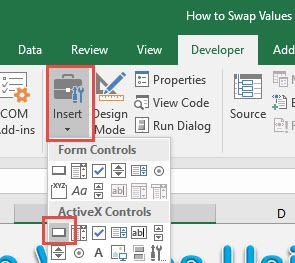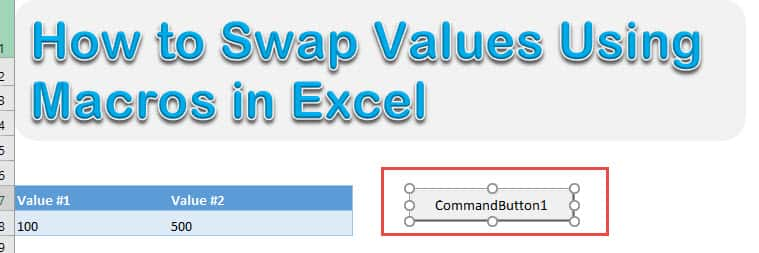STEP 2: Right click on your new button and select View Code.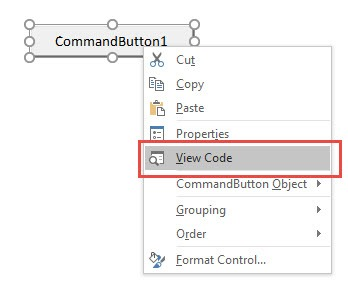STEP 3: Type in this code:

Dim container As Double

container = Range(“A8”).Value
Range(“A8”).Value = Range(“B8”).Value
Range(“B8”).Value = container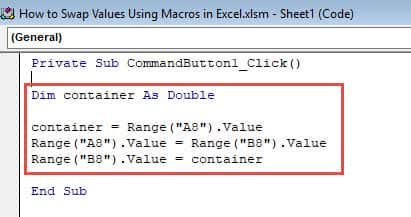Let us go through each line step by step:

Dim container As Double

This creates a new variable named container wherein we can store a numerical value inside. The double type means it can have a numerical value.

container = Range(“A8”).Value

Now we are getting the first value in Cell A8 and storing it into our container variable.

Range(“A8”).Value = Range(“B8”).Value

We will now copy the content of Cell B8 and paste it into Cell A8. First step done! So far both will have the value 500 in our example.

Range(“B8”).Value = container

Now the last step! Copy the content of the container variable into Cell B8. The swap is now complete!

STEP 4: Time to test it out! Make sure Design Mode is deselected.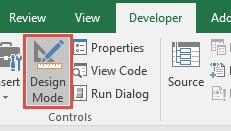STEP 5: Click on the button now and you will see the values get swapped!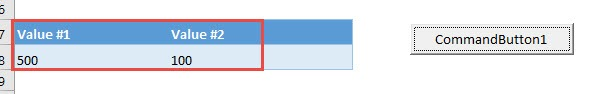Swap Values Using Macros in Excel